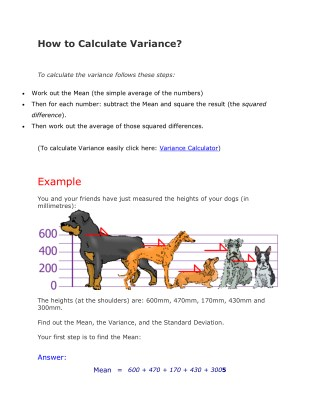DownloadDownload PresentationVariance Calculator - How to Calculate Variance?

# Variance Calculator - How to Calculate Variance?

Télécharger la présentation## Variance Calculator - How to Calculate Variance?

- - - - - - - - - - - - - - - - - - - - - - - - - - - E N D - - - - - - - - - - - - - - - - - - - - - - - - - - -
##### Presentation Transcript

1. How to Calculate Variance? To calculate the variance follows these steps: Work out the Mean (the simple average of the numbers) • Then for each number: subtract the Mean and square the result (the squared • difference). Then work out the average of those squared differences. • (To calculate Variance easily click here: Variance Calculator) Example You and your friends have just measured the heights of your dogs (in millimetres): The heights (at the shoulders) are: 600mm, 470mm, 170mm, 430mm and 300mm. Find out the Mean, the Variance, and the Standard Deviation. Your first step is to find the Mean: Answer: Mean=600 + 470 + 170 + 430 + 3005

2. =19705 =394 so the mean (average) height is 394 mm. Let's plot this on the chart: Now we calculate each dog's difference from the Mean: To calculate the Variance, take each difference, square it, and then average the result: Variance σ2 = 2062 + 762+ (−224)2 + 362+ (−94)25 = 42436 + 5776 + 50176 + 1296 + 88365 = 1085205 = 21704 So the Variance is 21,704 And the Standard Deviation is just the square root of Variance, so: Standard Deviation

3. σ= √21704 = 147.32... = 147(to the nearest mm) And the good thing about the Standard Deviation is that it is useful. Now we can show which heights are within one Standard Deviation (147mm) of the Mean: So, using the Standard Deviation we have a "standard" way of knowing what is normal, and what is extra large or extra small. Rottweilers are tall dogs. And Dachshunds are a bit short ... but don't tell them!